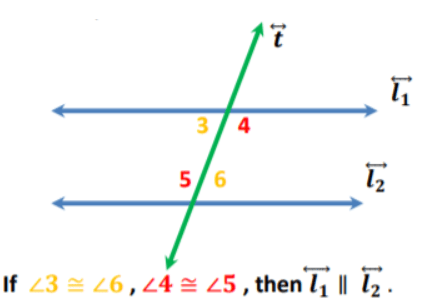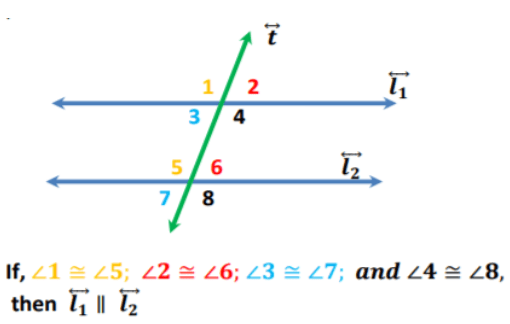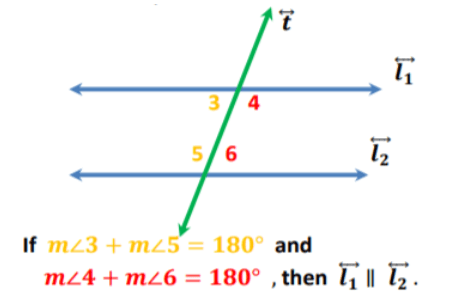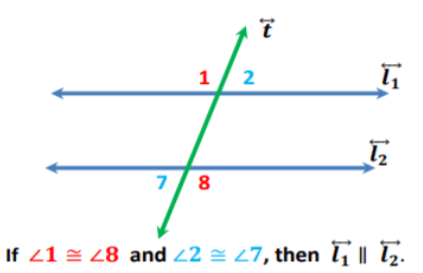# How to Prove Lines Are Parallel

In advanced geometry lessons, students learn how to prove lines are parallel. More specifically, they learn how to identify properties for parallel lines and transversals and become fluent in constructing proofs that involve two lines parallel or not, that are cut by a transversal.

For many students, learning how to prove lines are parallel can be challenging and some students might need special strategies to address difficulties. To help you out, we’ve compiled a list of awesome teaching strategies for your classroom. Read on and learn more.

## Teaching Strategies on How to Prove Lines Are Parallel

### Review Logic in Geometry and Proof

In your lesson on how to prove lines are parallel, students will need to be mathematically fluent in building an argument. They should already know how to justify their statements by relying on logic. That’s why it’s advisable to briefly review earlier knowledge on logic in geometry.

You can check out our article on this topic for more guidelines and activities, as well as this article on proving theorems in geometry which includes a step-by-step introduction on statements and reasons used in mathematical proofs.

You may also want to look at our article which features a fun intro on proofs and reasoning.

### Ways to Prove Lines Are Parallel

For starters, draw two parallel lines on the whiteboard, cut by a transversal. Remind students that a line that cuts across another line is called a transversal. If the line cuts across parallel lines, the transversal creates many angles that are the same.

Remind students that when a transversal cuts across two parallel lines, it creates 8 angles, which we can sort out in angle pairs. Point out that we will use our knowledge on these angle pairs and their theorems (i.e. the converse of their theorems) when proving lines are parallel.

More specifically, point out that we’ll use:

• the converse of the alternate interior angles theorem
• the converse of the corresponding angles theorem
• the converse of the same-side interior angles postulate
• the converse of the alternate exterior angles theorem#### Converse of the Alternate Interior Angles Theorem

Students are probably already familiar with the alternate interior angles theorem, according to which if the transversal cuts across two parallel lines, then the alternate interior angles are congruent, that is, they have exactly the same angle measure.

After you remind them of the alternate interior angles theorem, you can explain that the converse of the alternate interior angles theorem simply states that if two lines and a transversal form alternate interior angles that are congruent, then the two lines are parallel.

Draw two parallel lines and a transversal on the whiteboard to illustrate this:Explain that the alternate interior angles are represented by two angle pairs 3 and 6, as well as 4 and 5 with separate colors respectively. So if ∠ 3 is congruent to ∠ 6, and if ∠ 3 is congruent to ∠ 5, then the two lines are parallel.

#### Converse of the Corresponding Angles Theorem

Remind students that the corresponding angles theorem states that if the transversal cuts across two parallel lines, then the corresponding angles are congruent or equal in angle measure.

Now you can explain the converse of the corresponding angles theorem, according to which if two lines and a transversal form corresponding angles that are congruent, then the lines are parallel.

Draw two parallel lines and a transversal on the whiteboard to illustrate the converse of the corresponding angles theorem:Mark the angle pairs of corresponding angles with different colors respectively, as shown on the drawing. Explain that if ∠ 1 is congruent to ∠ 5, ∠ 2 is congruent to ∠ 6, ∠ 3 is congruent to ∠ 7 and ∠ 4 is congruent to ∠ 8, then the two lines are parallel.

#### Converse of the Same-side Interior Angles Postulate

Remind students that the same-side interior angles postulate states that if the transversal cuts across two parallel lines, then the same-side interior angles are supplementary, that is, their sum equals 180 degrees.

Now, explain that the converse of the same-side interior angles postulate states that if two lines and a transversal form same-side interior angles that are supplementary, then the two lines are parallel.

Draw two parallel lines and a transversal on the whiteboard to illustrate the converse of the same-side interior angles postulate:Mark the angle pairs of supplementary angles with different colors respectively, as shown on the drawing. Explain that if the sum of ∠ 3 equals 180 degrees and the sum of ∠ 4 and ∠ 6 equals 180 degrees, then the two lines are parallel.

#### Converse of the Alternate Exterior Angles Theorem

Remind students that the alternate exterior angles theorem states that if the transversal cuts across two parallel lines, then alternate exterior angles are congruent or equal in angle measure.

Now, point out that according to the converse of the alternate exterior angles theorem, if two lines and a transversal form alternate exterior angles that are congruent, then the two lines are parallel.

Draw two parallel lines and a transversal on the whiteboard to illustrate the converse of the alternate exterior angles theorem:Like in the previous examples, make sure you mark the angle pairs of alternate exterior angles with different colors. Explain to students that if ∠1 is congruent to ∠ 8, and if ∠ 2 is congruent to ∠ 7, then the two lines are parallel.

If you have the technical means in your classroom, you may also decide to complement your lesson on how to prove lines are parallel with multimedia material, such as videos. This free geometry video is a great way to do so.

The video contains simple instructions and examples on the converse of the alternate interior angles theorem, converse of the corresponding angles theorem, converse of the same-side interior angles postulate, as well as the converse of the alternate exterior angles theorem.

Start with a brief introduction of proofs and logic and then play the video. Pause and repeat as many times as needed. Prepare additional questions on the ways of proof demonstrated and end with a guided discussion.

## Activities for Proving Lines Are Parallel

### Pair Work

This is a simple activity that will help students reinforce their skills at proving lines are parallel. Introduce this activity after you’ve familiarized students with the converse of the theorems and postulates that we use in proving lines are parallel.

Prepare a worksheet with several math problems on how to prove lines are parallel. For instance, students are asked to prove the converse of the alternate exterior angles theorem using the two-column proof method. Include a drawing and which angles are congruent.

Divide students into pairs. Hand out the worksheets to each student and provide instructions. Students work individually to complete their worksheets. After 15 minutes, they review each other’s work and provide guidance and feedback.

## Before You Leave…

If you liked our teaching strategies on how to prove lines are parallel, and you’re looking for more math resources for kids of all ages, sign up for our emails to receive loads of free resources, including worksheets, guided lesson plans and notes, activities, and much more!

Feel free to also head over to our blog, where you’ll find plenty of free and awesome resources! And if you’d like to become a member, join us in the Math Teacher Coach community!